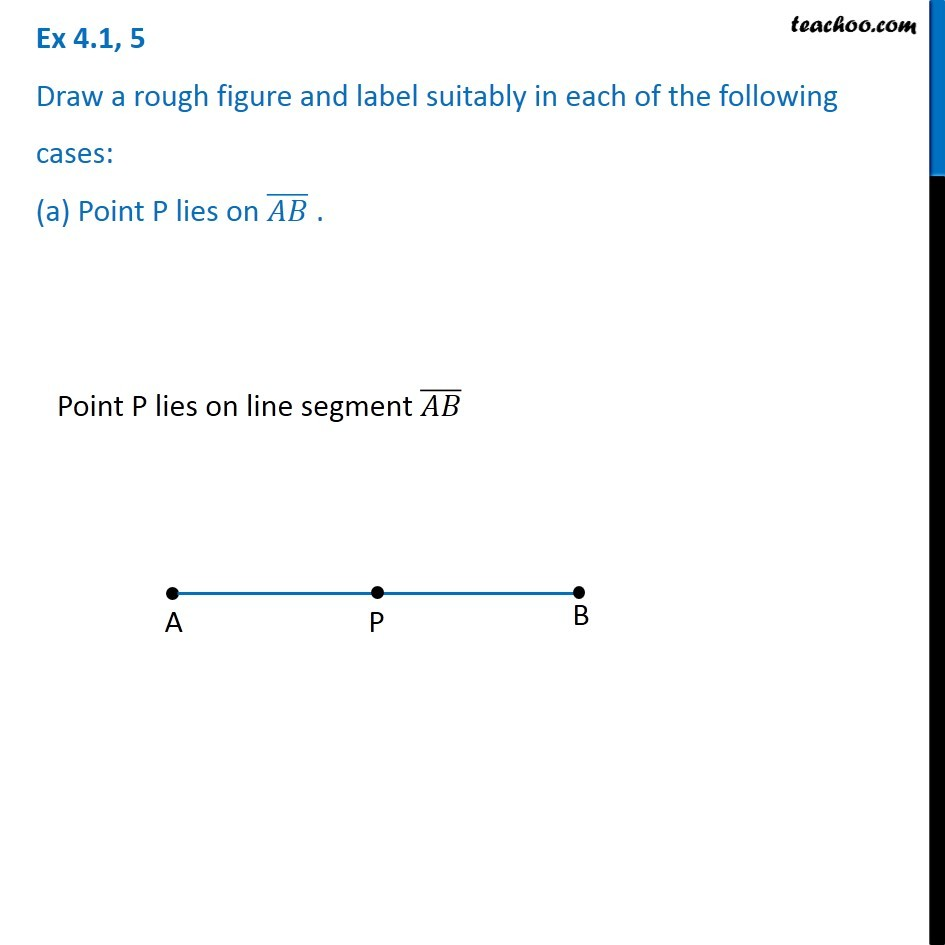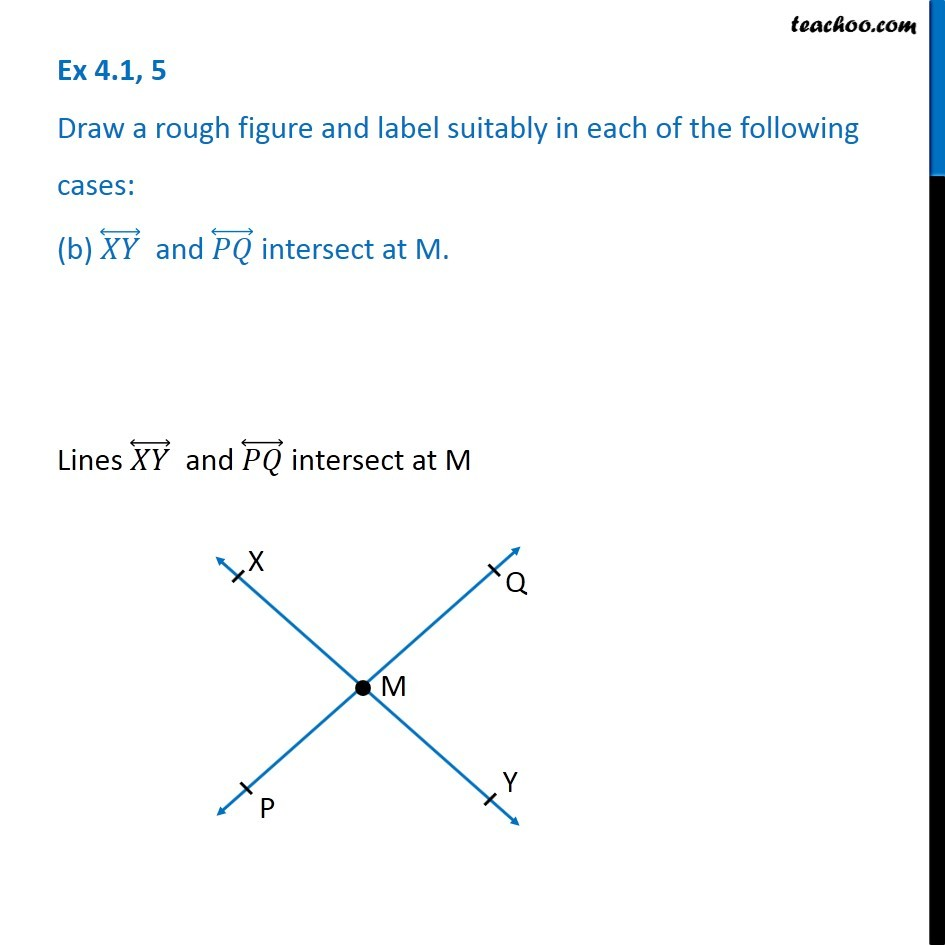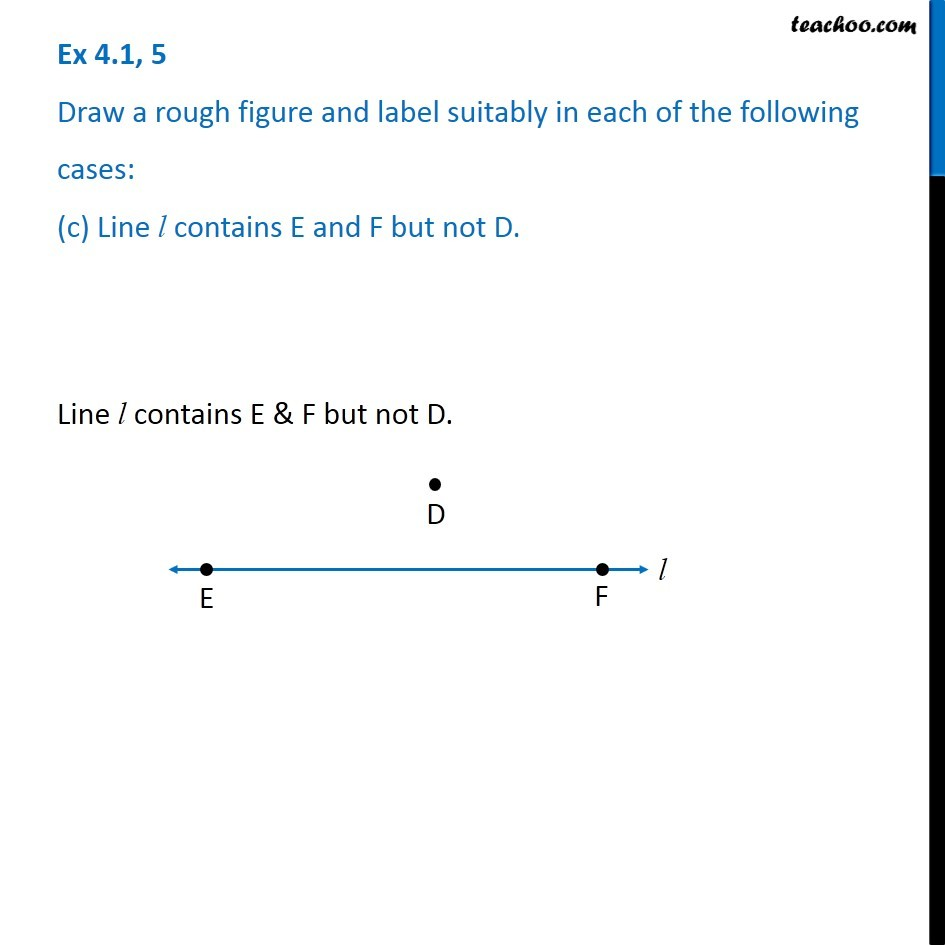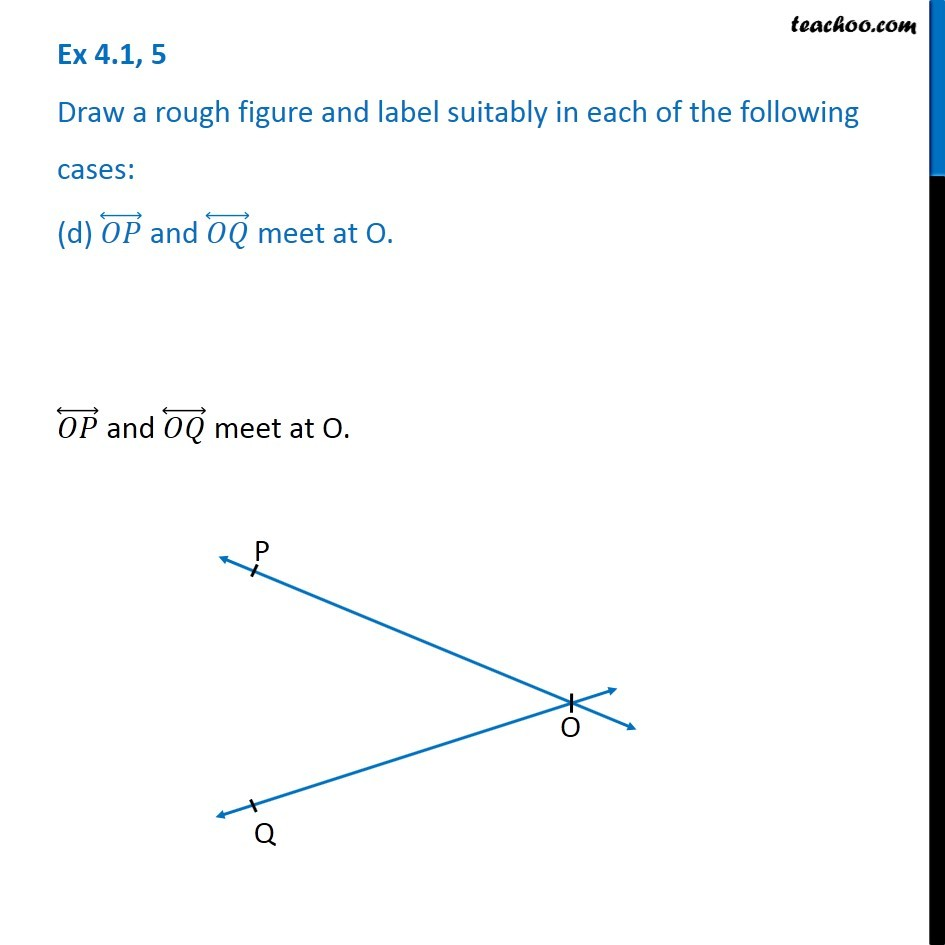Subscribe to our Youtube Channel - https://you.tube/teachoo

1. Chapter 4 Class 6 Basic Geometrical Ideas
2. Concept wise
3. Point, Line, ray, angle

Transcript

Ex 4.1, 5 Draw a rough figure and label suitably in each of the following cases: (a) Point P lies on (𝐴𝐵) ̅ . Point P lies on line segment (𝐴𝐵) ̅ Ex 4.1, 5 Draw a rough figure and label suitably in each of the following cases: (b) (𝑋𝑌) ⃡ and (𝑃𝑄) ⃡ intersect at M. Lines (𝑋𝑌) ⃡ and (𝑃𝑄) ⃡ intersect at M Ex 4.1, 5 Draw a rough figure and label suitably in each of the following cases: (c) Line l contains E and F but not D. Line l contains E & F but not D. Ex 4.1, 5 Draw a rough figure and label suitably in each of the following cases: (d) (𝑂𝑃) ⃡ and (𝑂𝑄) ⃡ meet at O. (𝑂𝑃) ⃡ and (𝑂𝑄) ⃡ meet at O.

Point, Line, ray, angle